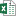# Convert Text to Time Values with Formulas

Bottom line: Learn how to convert times stored as text [## hours ## minutes ## seconds] to time values [h:mm:ss] that can be used for calculations and data analysis in Excel.

Skill level: Intermediate

## The Data Cleansing Challenge

This post is the first in a series on solutions to the data cleansing challenge I presented in a previous post. The challenge is to use any tools in Excel to convert the time/duration stored a text into a numeric value that can be formatted as a time.

The original data set contains a column of time/duration text that are in the following format.

`1 hour 27 minutes 15 seconds`

We need to convert it to a number in Excel with a time format of h:mm:ss that looks like the following.

`1:27:15`

## Formula based Solutions

There were a ton of awesome solutions submitted on the original post and YouTube video. Thanks again if you submitted a solution!

The most popular solutions used functions and formulas to extract the times values from the text, and convert it to a time.

In the next posts we'll look at how to use Power Query and VBA UDF's to do this conversion.Text To Time Challenge - Formula Solutions.xlsx (326.5 KB)

The file uses Excel Tables. Checkout my post and video on a beginner's guide to Excel Tables if you aren't familiar with this awesome Excel feature yet.

## Solution #1: Text Functions

In the video below I walk through how to solve the challenge with text functions. The text functions (MID and SEARCH) are used to find and extract the numbers for each time period (hours, minutes, seconds).

### Explanation of the formula

The formula to extract each time increment looks like the following.

`=IFERROR(VALUE(MID(\$C2,MAX(SEARCH(E\$1,\$C2)-3,1),2)),0)`

In the video I explain each component and walk through how to write the formula.

Here is a brief explanation of what each function does in the formula.

• SEARCH – find text within a string of text and return the number of the starting character.
• MID – return/extract a portion of the text based on the starting charcter number and length of characters.
• MAX – return a 1 if SEARCH returns a zero for the starting character. Used in place of an IF function.
• VALUE – convert the text returned by MID to a numeric value.
• IFERROR – handles the error returned by SEARCH if the find_text is not found. Return a zero to denote that the time period (hr, min, sec) does not exist in the text.

## Solution #2: SUMPRODUCT Function

The next solution uses the SUMPRODUCT function to convert all time periods to seconds. Thanks to Laura and Wong for sharing this technique. Wong has a full article on this formula over at wmfexcel.com.

### Explanation of the formula

The formula uses the same basic text extraction technique from solution #1 above.

However, it also uses arrays within the SUMPRODUCT formula. This allows us to do the extraction for each time period within one SEARCH function.

`=SUMPRODUCT(VALUE(IFERROR(MID(0&C2,SEARCH({"h","m","sec"},0&C2)-3,2),{0,0,0})),{3600,60,1})/86400`

The {“h”,”m”,”sec”} is an array, or list of values, that is used in the find_text argument of SEARCH. This returns the results of the search in an array back to the SUMPRODUCT function.

So the text time of 10 hours 39 minutes 40 seconds would return the following array to the MID(SEARCH()) portion of the formula.

`{10,39,40}`

You can think of the SEARCH function as calculating three separate times to return those results.

The second array in SUMPRODUCT is the amount of seconds in each time period {hr, min, sec}.

`{3600,60,1}`

There are 3,600 seconds in an hour (60*60), 60 seconds in a minute, and 1 second in a second.

SUMPRODUCT multiplies each of the values together and then sums them up.

`(10*3600)+(39*60)+(40*1)`

This returns the total number of seconds. That number is divided by the number of seconds in a day 86,400 = (24*60*60).

The result is a decimal number or the fraction of a whole day. Each day is Excel is represented by a whole number. Checkout my article on date data types and the calendar system in Excel to learn more.

### What if the duration greater than 24 hours?

The advantage of the SUMPRODUCT solution is that it can handle times that are over 24 hours. Since it is returning the number of seconds, anything over 86,400 seconds will still return a decimal number.

We can use the following format to display the number of days, hours, minutes, and seconds.

`d.h:mm:ss`

The SUMPRODUCT formula is a very robust solution, and it's super nifty. 👍

## Conclusion

There are a lot of different ways to solve this problem with formulas. In this post we looked at two different formulas to extract the time increments and convert them to time values.

In the next posts we will look at solutions with Power Query and VBA UDF's (User Defined Functions).

•Peter says:

Is there a way to do the reverse?
Convert Time Values to Text

eg. converting 1:15:10 to 1 hour 15 minutes 10 seconds

•MF says:

Thanks for mentioning.
Feeling honored

•David N says:

Just be advised that the SUMPRODUCT solution is susceptible to Excel’s rounding problem for lengthy decimals, such that right answers to our eyes are occasionally not “right answers” to Excel. The 4:43:23 result for row 979 is an example. For more details, see my reply to Toni on page 2 of the comments for the original post, and note that this variation from Laura and Wong only results in 8 near misses instead of the 14 from Toni’s approach.

Generic filters
Exact matches only
Filter by Custom Post TypeLearn 10 great Excel techniques that will wow your boss and make your co-workers say, "how did you do that??"